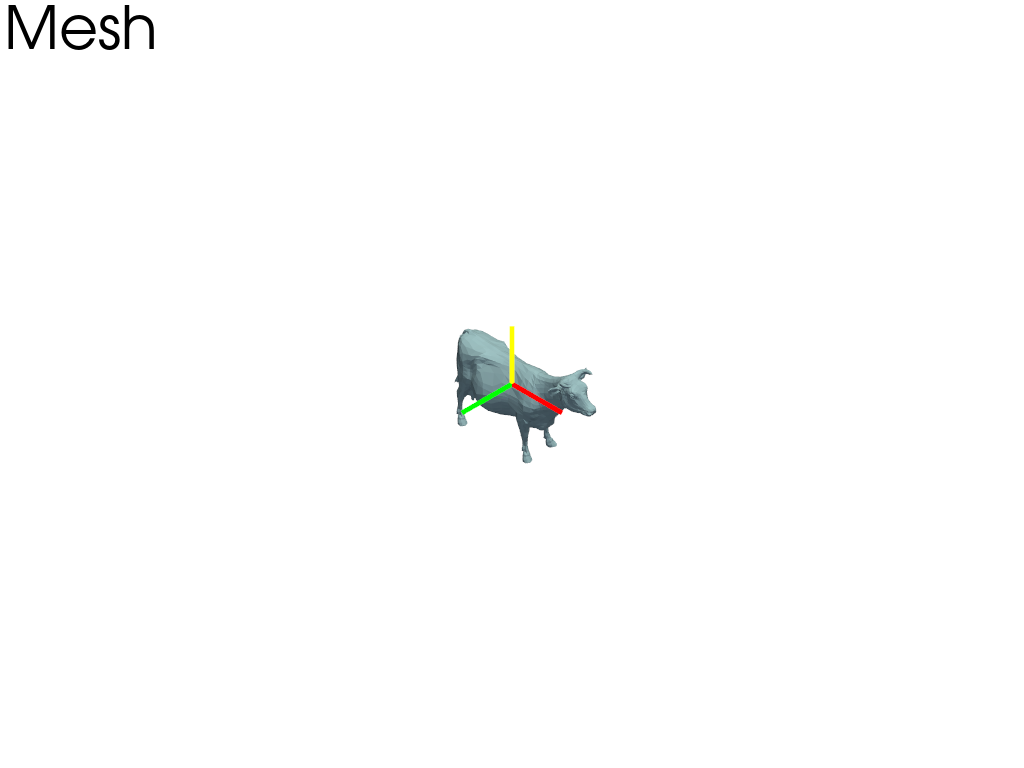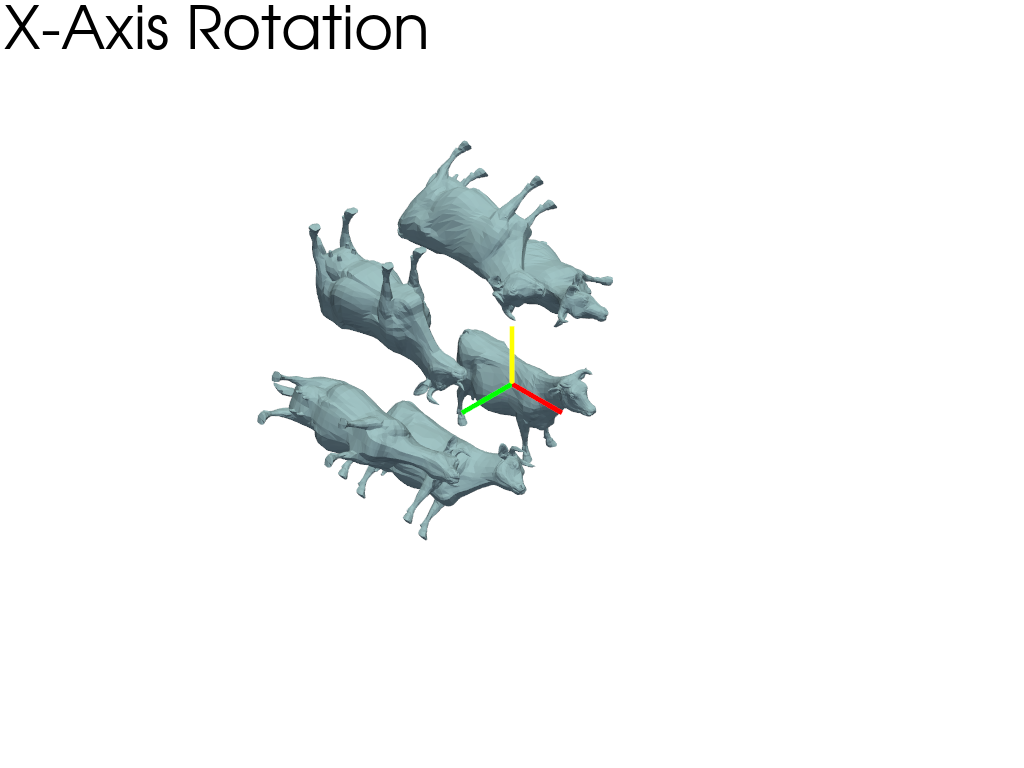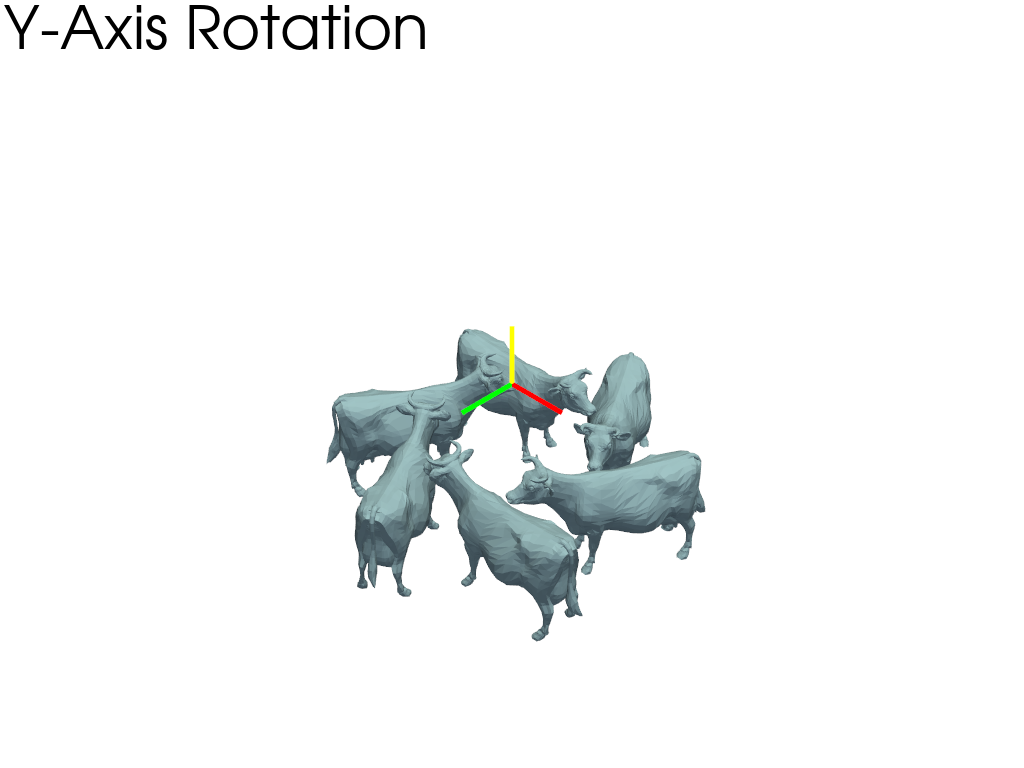# Rotations#

Rotations of a mesh about its axes. In this model, the x axis is from the left to right; the y axis is from bottom to top; and the z axis emerges from the image. The camera location is the same in all four images.

```import pyvista as pv
from pyvista import examples
```

## Define camera and axes#

Define camera and axes. Setting axes origin to `(3.0, 3.0, 3.0)`.

```mesh = examples.download_cow()
mesh.points /= 1.5  # scale the mesh

camera = pv.Camera()
camera.position = (30.0, 30.0, 30.0)
camera.focal_point = (5.0, 5.0, 5.0)

axes = pv.Axes(show_actor=True, actor_scale=2.0, line_width=5)
axes.origin = (3.0, 3.0, 3.0)
```

## Original Mesh#

Plot original mesh. Add axes actor to Plotter.

```p = pv.Plotter()

p.camera = camera

p.show()
```## Rotation about the x axis#

Plot the mesh rotated about the x axis every 60 degrees. Add the axes actor to the Plotter and set the axes origin to the point of rotation.

```p = pv.Plotter()

p.camera = camera

for i in range(6):
rot = mesh.rotate_x(60 * i, point=axes.origin, inplace=False)

p.show()
```## Rotation about the y axis#

Plot the mesh rotated about the y axis every 60 degrees. Add the axes actor to the Plotter and set the axes origin to the point of rotation.

```p = pv.Plotter()

p.camera = camera

for i in range(6):
rot = mesh.rotate_y(60 * i, point=axes.origin, inplace=False)

p.show()
```## Rotation about the z axis#

Plot the mesh rotated about the z axis every 60 degrees. Add axes actor to the Plotter and set the axes origin to the point of rotation.

```p = pv.Plotter()

p.camera = camera

for i in range(6):
rot = mesh.rotate_z(60 * i, point=axes.origin, inplace=False)

p.show()
```## Rotation about a custom vector#

Plot the mesh rotated about a custom vector every 60 degrees. Add the axes actor to the Plotter and set axes origin to the point of rotation.

```p = pv.Plotter()

p.add_text("Custom Vector Rotation", font_size=24)
p.camera = camera
for i in range(6):
rot = mesh.copy()
rot.rotate_vector(vector=(1, 1, 1), angle=60 * i, point=axes.origin)

p.show()
```Out:

```/home/alex/python/pyvista/pyvista/core/pointset.py:335: PyvistaDeprecationWarning: You did not specify a value for `inplace` and the default value will be changing to `False` in future versions for point-based meshes (e.g., `PolyData`). Please make sure you are not assuming this to be an inplace operation.